# MCQs on Power Systems

##### Page 13 of 67. Go to page 1 2 3 4 5 6 7 8 9 10 11 12 13 14 15 16 17 18 19 20 21 22 23 24 25 26 27 28 29 30 31 32 33 34 35 36 37 38 39 40 41 42 43 44 45 46 47 48 49 50 51 52 53 54 55 56 57 58 59 60 61 62 63 64 65 66 67
01․ In a long transmission line, if we increase the length of the line, the parameters A and B respectively
increases and increases
decreases and decreases
decreases and increases
increases and decreases

For long transmission lines A = coshγl B = Zc sinhγl C = sinhγl/Zc D = coshγl Where, γ = Propagation constant = √(ZY) l = Length of the line Zc = Characteristic impedance Therefore, by increasing of length, A and D will decreases and B and C will increases.

02․ Power transmission lines are transposed to reduce
skin effect
proximity effect
ferranti effect

If power line and telecommunication lines are running close to each other, the current flowing in the power line produces magnetic flux linkage with the communication line conductor induces an emf in the telecommunication line conductor.This is called electro magnetic induction. Similarly due to earth effect electric field is produced by the charges of the earth induces a voltage in between the conductors of the telecommunication lines. This is called electro static induction. This both electro magnetic induction and electro static induction produces the voltage between the telecommunication line conductors which causes interference to the telecommunication signals which is called radio interference. To reduce this radio interference in the telecommunication line, either power line or both power and telecommunication lines are transposed at regular intervals of length the transmission line.

03․ Find the propagation constant, if impedance is 10Ω and admittance is 10 â„§?
100
1
10
5

Propagation constant (γ): With a voltage and current signals travelling or propagating through the transmission line, due to resistance and conductance attenuation occurs and due to inductance and capacitance phase shift occurs. This is expressed by propagation constant (γ). Propagation constant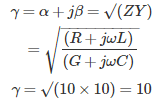04․ Attenuation occurs due to
resistance
inductance
conductance
both 1 and 3

Attenuation constant (α): Due to resistance and conductance components of transmission line attenuation or decrease of voltage and current signals are occurs. It is measured in dB or neper.

05․ Phase shift occurs due to which of the following components?
inductance
capacitance
both 1 and 2
conductance

Phase shift constant (β): Due to inductance and capacitance components phase shift of voltage or current signals occurs in the transmission line. It is measured in degrees or radians.

06․ Find the characteristic impedance of a transmission line, if impedance is 16 Ω and admittance is 0.1 mâ„§?
400 Ω
160 Ω
200 Ω
320 Ω

Characteristic impedance (Zc): It will give the relation between voltage and current flowing through the transmission line which are depending on the transmission line parameters, i.e., R,G,L and C. Characteristic impedance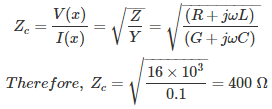07․ A loss less transmission line has a length of 600 km, find the phase shift of voltage signal?
90°
42°
36°
45°

Propagation constant (γ): With a voltage and current signals travelling or propagating through the transmission line, due to resistance and conductance attenuation occurs and due to inductance and capacitance phase shift occurs. This is expressed by propagation constant (γ). Propagation constant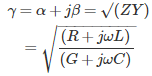Where, α = Attenuation constant β = Phase shift constant For loss less line, R = G = 0 Therefore, α = 0,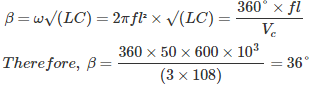Where, Vc = velocity of signal l = length of the line

08․ For loss less line, find the ratio of length to the wave length of a signal for a phase shift of 0.05 radians?
8 × 10³
7.95 × 10-3
7.95 × 10³
2 × 10-3

Propagation constantWhere, α = Attenuation constant β = Phase shift constant For loss less line, R = G = 0 Therefore, α = 0,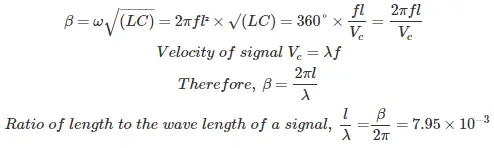09․ A transmission line is operating under no load has ABCD parameters B = 100∠90° has a phase shift of 0.2 rad, how much shunt reactor is required for compensation of ferranti effect?
2 mH
15.9 mH
15.9 H
2 H

Shunt reactance Xsh = B/(1-A) A = 1 + YZ/2 = 1 + (G+jωC)(R+jωL)/2 For loss less line, R = G = 0 Therefore, A = 1 - ω²LC/2 A = 1 - β²/2 Where, β = Phase shift = ω√(LC) A = 1 - 0.2²/2 = 0.98 Shunt reactance Xsh = 100∠90°/(1 - 0.98) = 5 kΩ L = 5*10³/(2π*50) = 15.9 H

10․ A transmission line has a surge impedance of 400 Ω for a length of the line of 200 km. If the length of line is increased to 400 km, then its surge impedance is
400 Ω
800 Ω
200 Ω
0 Ω

Characteristic impedance (Zc): It will give the relation between voltage and current flowing through the transmission line which are depending on the transmission line parameters, i.e., R,G,L and C.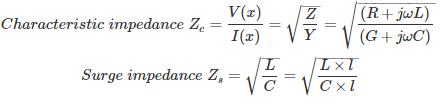Therefore, surge impedance is independent of length of the line.

<<<1112131415>>>